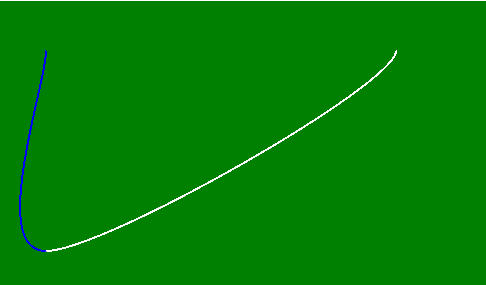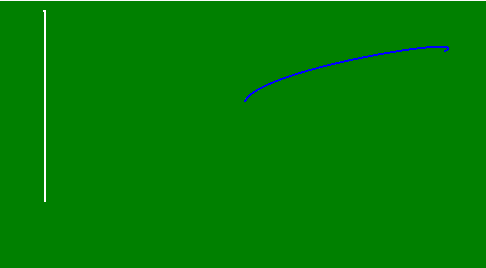# p5.js | curve() function

• Last Updated : 17 Jan, 2020

The curve() function is used to draws a curved line between two points given in the middle four parameters on the screen. The first two and last two parameters are used as a control point.

Syntax:

`curve( x1, y1, x2, y2, x3, y3, x4, y4 )`

or

`curve( x1, y1, z1, x2, y2, z2, x3, y3, z3, x4, y4, z4 )`

Parameters:

ValueDescription
x1It is used to hold the x-coordinate of beginning control point.
y1It is used to hold the y-coordinate of beginning control point.
z1It is used to hold the z-coordinate of beginning control point.
x2It is used to hold the x-coordinate of first point.
y2It is used to hold the y-coordinate of first point.
z2It is used to hold the z-coordinate of first point.
x3It is used to hold the x-coordinate for second point.
y3It is used to hold the y-coordinate for second point.
z3It is used to hold the z-coordinate for second point.
x4It is used to hold the x-coordinate of ending control point.
y4It is used to hold the y-coordinate of ending control point.
z4It is used to hold the z-coordinate of ending control point.

Below examples illustrate the curve() function in CSS:

Example 1:

 `function setup() { ``   ` `    ``// Create canvas of given size``    ``createCanvas(500, 300); ``   ` `    ``// Set the background of canvas``    ``background('green'); ``} `` ` `function draw() { ``     ` `    ``// Use noFill() function to not fill the color``    ``noFill();``   ` `    ``// Set the stroke color``    ``stroke('white');``   ` `    ``// Use curve() function to create curve``    ``curve(50, 50, 400, 50, 50, 250, 50, 50);`` ` `    ``// Set the stroke color``    ``stroke('blue');``   ` `    ``// Use curve() function to create curve``    ``curve(400, 50, 50, 250, 50, 50, 50, 50); ``} `

Output:Example 2:

 `function setup() { ``   ` `    ``// Create canvas of given size``    ``createCanvas(500, 300); ``   ` `    ``// Set the background of canvas``    ``background('green'); ``} `` ` `function draw() { ``     ` `    ``// Use noFill() function to not fill the color``    ``noFill();``   ` `    ``// Set the stroke color``    ``stroke('white');``   ` `    ``// Use curve() function to create curve``    ``curve(50, 50, 50, 200, 50, 10, 50, 250, 150, 50, 50, 50);`` ` `    ``// Set the stroke color``    ``stroke('blue');``   ` `    ``// Use curve() function to create curve``    ``curve(50, 200, 450, 50, 250, 100, 350, 250, 250, 450, 450, 400); ``} `

Output:Online editor: https://editor.p5js.org/
Environment Setup: https://www.geeksforgeeks.org/p5-js-soundfile-object-installation-and-methods/

My Personal Notes arrow_drop_up